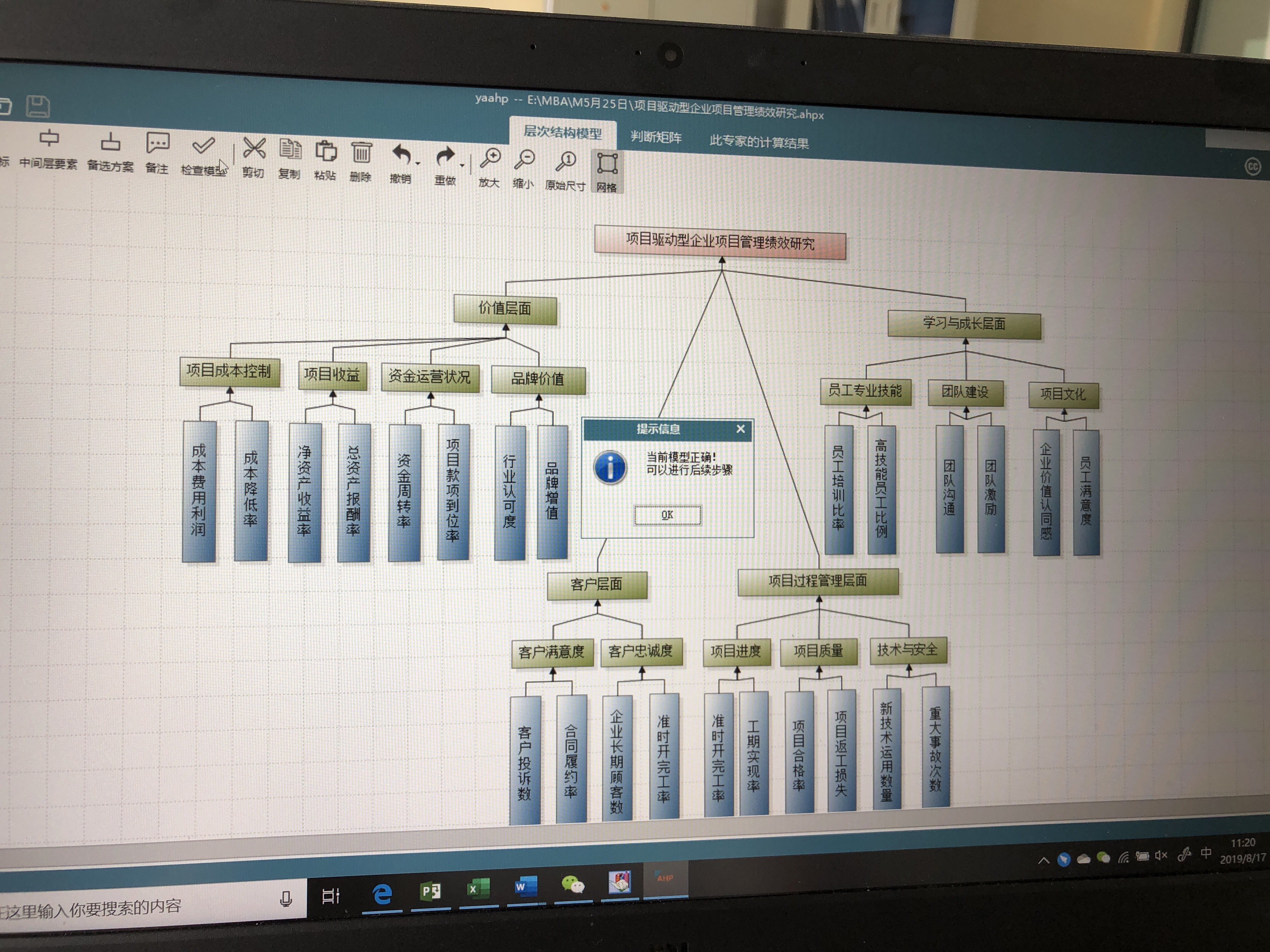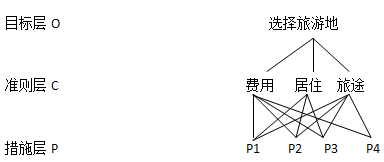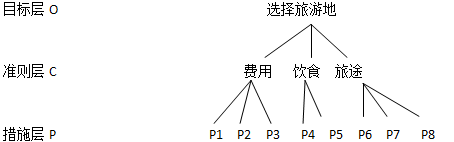• 论文研究-层次分析法判断矩阵不一致性的形成机理和一种修正方法.pdf, 本文对层次分析法判断矩阵不一致性的形成机理进行了探讨, 分析了构成判断误差及影响判断矩阵不满足...
• 建立判断矩阵 层次分析法的一个重要特点就是用两两重要性程度之比的形式表示出两个方案的相应重要性程度等级。 4.计算层次单排序的权向量和一致性检验 对于每一个成对比较阵计算最大特征根及对应特征向量，利用一致...
一、引言
供应商管理，是在新的物流与采购经济形势下，提出的管理机制。供应链管理环境下的客户关系是一种战略性合作关系，提倡一种双赢机制。从传统的非合作性竞争走向合作性竞争、合作与竞争并存是当今企业关系发展的一个趋势。因此供应商选择对企业采购与供应工作具有直接的关键的影响。一个好的供应商能够在物料或服务的质量、成本等各个方面满足企业的需求，一个不合格的供应商在这些方面会给企业带来非常严重的问题与后果。无论是在传统的采购与供应管理中，还是在现在的供应链管理中，供应商选择都是关键环节，选择一个卓越的供应商，是企业的一项关键职能。
供应商选择的一般原则是：资金力量较强，产品质量较好，价格合理，服务质量好。所以本文的评价指标主要考虑质量、价格、交货、服务和提前期这五个指标。对这五个评价指标的具体描述如下：
产品质量：指供应商的产品满足企业需求的程度。一般用合格产品占总产品的比重计算，该指标越大越好，是“效益”型指标。
产品价格：指企业采购的每一单位产品所付出的成本，该指标越小越好，是“成本”型指标。
交货：指供应商及时满足企业定单的程度。一般用及时交货的定单数占总定单数的比例来表示，该指标值越大越优，是“效益”型指标。
服务：指供应商在交货后在产品安装、维护等方面满足企业的程度，该指标值越大越好，为“效益”型指标。
交货提前期：指企业发出定单到收到定货之间的时间，对于需求方来说，交货提前期越短越好，是“成本”型指标。
二、方法介绍
层次分析法首先将整个系统划分为目标、准则和方案三个层次，然后对方案进行相互比较，运用矩阵形式判断作相对评价，最后进行综合评价，排出各方案的优劣次序。具体做法可分为五个步骤：
1.明确目标
2.建立层次结构
在深入分析实际问题的基础上，将有关的各个因素按照不同属性自上而下地分解成若干层次，同一层的诸因素从属于上一层的因素或对上层因素有影响，同时又支配下一层的因素或受到下层因素的作用。最上层为目标层，通常只有1个因素，最下层通常为方案或对象层，中间可以有一个或几个层次，通常为准则或指标层。当准则过多时应进一步分解出子准则层。
3.建立判断矩阵
层次分析法的一个重要特点就是用两两重要性程度之比的形式表示出两个方案的相应重要性程度等级。
4.计算层次单排序的权向量和一致性检验
对于每一个成对比较阵计算最大特征根及对应特征向量，利用一致性指标、随机一致性指标和一致性比率做一致性检验。若检验通过，特征向量(归一化后)即为权向量：若不通过，需重新构追成对比较阵。
5.进行总层次排序
计算最下层对目标的组合权向量，并根据公式做组合一致性检验，若检验通过，则可按照组合权向量表示的结果进行决策，否则需要重新考虑模型或重新构造那些一致性比率较大的成对比较阵。
应用这种方法，决策者通过将复杂问题分解为若干层次和若干因素，在各因素之间进行简单的比较和计算，就可以得出不同方案的权重，为最佳方案的选择提供依据。
三、建立模型
1.明确目标：选择理想的供应商。
2.建立层次结构模型：该结构图包括目标层，准则层，方案层。
4.计算层次单排序的权向量：W=（0.263，0.475，0.005，0.090，0.110）。CR=0.016＜0.1通过一致性验证。
同样求第3层(方案)对第2层每一元素(准则)的权向量：
w1=(0.595，0.277，0.129)，w2=（0.082，0.236，0.682），w3=（0.429，0.429，0.142），w4=（0.633，0.193，0.175），w5=（0.166，0.166，0.668）。CR1=0.05，CR2=0.002,CR3=0，CR4=0.009，CR5=0，均＜0.1，通过一致性检验。
5.进行总层次排序
P1对总目标的权值为：0.595×0.263+0.082×0.475+0.429×0.055+0.663×0.099+0.166×0.110=0.3同理P2、P3 对总目标的权值分别为：0.246，0.456 决策层对总目标的权向量为：{0.3，0.246，0.456}，CR=0.015＜0.1层次总排序通过一致性检验。因此最佳供应商为P3。
四、小结
本文运用层次分析法对供应链管理中的供应商选择进行了评价，充分考虑了供应商的质量、成本、交货、服务和提前期，综合考虑各个供应商的优劣势，以及该层对上层的影响，选择了一个最优的供应商。随着供应链思想的普遍应用，供应商的选择显得越来越重要，因此本文具有一定的现实意义。
文末资源：层次分析法常用软件，破解版yaahp软件，中间层个数可达99个，方案层个数可达99个，免费版的中间层、方案层个数只有3个哦，内含教程，永久使用：（浏览器输入/点击网址获取）
层次分析法之yaahp软件，中间层、方案层99个，内含教程，提供技术支持！
https://k.ruyu.com/l8veTM3e
下图是我做的架构：展开全文• 层次分析法matlab源程序，用于一致性检验，特征值、特征向量计算
• 讲解了如何利用Matlab求解层次分析法判断矩阵的权向量，并进行一致性判断。
• 论文研究- 如何增加层次分析法判断矩阵的一致性.pdf, 一、前言 美国运筹学家T.L.Saaty教授提出的层次分析法(AHP)已在我国用于许多决策研究中,但在实际应用时,参加评判的专家或决策者对判断矩阵的构造常感困难。...
• 层次分析法判断矩阵的填写方法、一致性检验的步骤、以及根据判断矩阵计算权重的方法

目录：

准则层判断矩阵怎么填写
方案层判断矩阵怎么填写
关于判断矩阵和一致矩阵的知识点补充
一致性检验的步骤
怎样通过判断矩阵去计算权重（三种方法），及相应的代码示例

准则层判断矩阵的填写：
填写准则层判断矩阵的目的是确定各准则（指标）所占的比重，填写好层次分析表的指标权重列，例如在选择最佳旅游地问题的指标景色、花费、居住、饮食、交通各自占比是多少，后续可以通过这些指标占比计算出每一个可选方案的总分。填表的方法是依据标度表，两两比较指标的重要程度，只需要比较10次就可以完成准则层判断矩阵的填写

方案层判断矩阵的填写
填写方案层判断矩阵的目的是给出，对于某一特定指标，它在各个可选方案的具体得分是多少，也就是给出层次分析表的每一横行的数据。方法是依据标度表，填写好判断矩阵。有几个评价指标，就需要填多少此方案层判断矩阵。知识点补充：
判断矩阵（正互反矩阵）

首先判断矩阵一定是一个方阵
判断矩阵每一个数据 Aij表示与指标 j相比 i的重要程度
当 i=j 时，两个指标相同，因此同等重要，记为1，因此判断矩阵的对角线元素为1
每一个元素均大于零，且 Aij * Aji=1

在层次分析法中，我们构造的矩阵的均为判断矩阵
一致矩阵

矩阵首先满足判断矩阵的所有特点
若判断矩阵满足 Aij * Ajk = Aik,直观的看就是矩阵的各行（各列）成倍数关系

注意点：在使用判断矩阵求权重之前，必须对其进行一致性检验

一致性检验的步骤：
第一步：计算一致性指标CI
$CI\,\,=\,\,\frac{\lambda _{\max}-n}{n-1}$
第二步：查找对应的平均随机一致性指标RI第三步：计算一致性比例CR
$CR\,\,=\,\,\frac{CI}{RI}$
判断：如果CR<0.1,则可认为判断举证的一致性可以接受；否则需要对判断矩阵进行修改
一致性检验的MATLAB代码如下：
disp('请输入判断矩阵A')
A=input('A=');
[n,n] = size(A);
[V,D] = eig(A);%求出矩阵A的特征值和特征向量
Max_eig = max(max(D));%找到矩阵A的最大特征值
% 下面是计算一致性比例CR的环节 %
CI = (Max_eig - n) / (n-1);
RI=[0 0.0001 0.52 0.89 1.12 1.26 1.36 1.41 1.46 1.49 1.52 1.54 1.56 1.58 1.59];
%注意哦，这里的RI最多支持 n = 15
% 这里n=2时，一定是一致矩阵，所以CI = 0，我们为了避免分母为0，将这里的第二个元素改为了很接近0的正数
CR=CI/RI(n);
disp('一致性指标CI=');disp(CI);
disp('一致性比例CR=');disp(CR);
if CR<0.10
disp('因为CR<0.10，所以该判断矩阵A的一致性可以接受!');
else
disp('注意：CR >= 0.10，因此该判断矩阵A需要进行修改!');
end



通过判断矩阵求权重
方法一、算数平均法求权重
第一步：将判断矩阵按照列归一化（每一个元素除以器所在列的和）
第二步：将归一化的各列相加（按行求和）
第三步：将相加后得到的向量中的每个元素除以n即可得到权重向量
具体数学表达：
假设判断矩阵为下面这个矩阵A：
$A=\left[ \begin{matrix} a_{11}& a_{12}& \cdots& a_{1n}\\ a_{21}& a_{22}& \cdots& a_{2n}\\ \vdots& \vdots& \ddots& \vdots\\ a_{n1}& a_{n2}& \cdots& a_{nn}\\ \end{matrix} \right]$
那么算数平均法求得的权重向量为：
$w_i=\frac{1}{n}\sum_{j=1}^n{\frac{a_{ij}}{\sum_{k=1}^n{a_{kj}}}}\,\,$
MATLAB代码如下：
disp('请输入判断矩阵A')
A=input('A=');
[n,n] = size(A);

Sum_A = sum(A);   %sum函数默认是对矩阵的每一列进行累加，即按行求和
SUM_A = repmat(Sum_A,n,1);    %将Sum_A这个行向量，重复n行，重复一列
Stand_A = A ./ SUM_A;         %将矩阵A归一化，即每一个元素除以其所在列的和

disp('算术平均法求权重的结果为：');
disp(sum(Stand_A,2)./n)      %把归一化的矩阵的每一行累加，然后除以n,得到权重

方法二、几何平均法求权重
第一步：将A元素按照行相乘得到一个新的列向量
第二步：将新的列向量的每个分量开n次方
第三步：对该列向量进行归一化即可得到权重向量
假设判断矩阵为下面这个矩阵A：
$A=\left[ \begin{matrix} a_{11}& a_{12}& \cdots& a_{1n}\\ a_{21}& a_{22}& \cdots& a_{2n}\\ \vdots& \vdots& \ddots& \vdots\\ a_{n1}& a_{n2}& \cdots& a_{nn}\\ \end{matrix} \right]$
那么几何平均法求得的权重向量为：
$w_i=\frac{\left( \prod_{j=1}^n{a_{ij}} \right) ^{\frac{1}{n}}}{\sum_{k=1}^n{\left( \prod_{j=1}^n{a_{kj}} \right) ^{\frac{1}{n}}}},\left( i=1,2,…\text{，}n \right)$
注意：每一种方法求得的权重和应该为1，由于四舍五入导致的误差可以忽略，一般结果保留四位小数
MATLAB代码如下：
disp('请输入判断矩阵A')
A=input('A=');
[n,n] = size(A);  %获得矩阵A的行和列的大小

Prduct_A = prod(A,2);       %把矩阵A的每一行累乘，即按照列累乘
Prduct_n_A = Prduct_A .^ (1/n);   %将新的列向量的每个分量开n次方
disp('几何平均法求权重的结果为：');
disp(Prduct_n_A ./ sum(Prduct_n_A))  %对该列向量进行归一化即可得到权重向量

方法三、特征值法求权重（常用）
知识点提醒：一致矩阵有一个特征值为n,其余特征值均为0，并且当矩阵的特征值为n时，其对应的特征向量为
$k\left[ \frac{1}{a_{11}},\frac{1}{a_{12}},…,\frac{1}{a_{1n}} \right] ^T,\left( k\ne 0 \right)$
第一步：求出矩阵A的最大特征值和以及其对应的特征向量
第二步：对求出的特征向量进行归一化即可得到所求的权重
MATLAB代码如下：
disp('请输入判断矩阵A')
A=input('A=');

%求矩阵A的全部特征值，构成对角阵D，求A的特征向量构成V的列向量（V的每一列都是D中与之相同列的特征值的特征向量）
[V,D] = eig(A);
Max_eig = max(max(D));   %求出矩阵A的最大的特征值
[r,c]=find(D == Max_eig , 1);  %返回最大特征值所在的行和列，其中C记录所在列
disp('特征值法求权重的结果为：');
disp( V(:,c) ./ sum(V(:,c)) )  %对最大特征值对应的特征向量进行归一化处理

友情提示：在比赛当中，建议三种方法全部列出来，但仅适用特征值法求得的权重结果进行计算

更多有关与层次分析法的经典获奖论文，以及建模论文模板等资料关注公众号，回复，“层次分析法”，即可免费领取！！！展开全文• 构造判断矩阵的讲解(层次分析法)讲解判断矩阵和层次分析的关系。以及MATLAB的一些应用。
• 在AHP模型中，请一组专家对某一属性进行评价，根据专家的判断能力，给出判断力权值，再通过适当的数学处理方法，可得最优判断矩阵，并根据残差来确定所求判断矩阵是否达到精度要求，若不符合精度要求，则需返还给...
• C#.net层次分析法中的判断矩阵构建，包括一致性检验，封装成类后，调用很方便
•   一个经典的层次分析法的结构图如下：   准则层的每个指标均与措施层的每个指标都具有联系和比重。在措施层的维度较多时，可能出现的情况是 准则层判断矩阵 费用 饮食 旅途 费用 1 3 5 饮食 1/3 1...
  一个经典的层次分析法的结构图如下：准则层的每个指标均与措施层的每个指标都具有联系和比重。在措施层的维度较多时，可能出现的情况是准则层判断矩阵

费用
饮食
旅途

费用
1
3
5

饮食
1/3
1
1

旅途
1/5
1
1

方案层判断矩阵分别为

P1
P2
P3

P1
1
1/3
1/3

P2
3
1
1

P3
3
1
1

P4
P5

P4
1
1/5

P5
5
1

P6
P7
P8

P6
1
3
1

P7
1/3
1
1

P8
1
1
1

上面的情况是最极端的情况（措施层的指标仅与决策层的一个指标相关），而本文针对任何形式的方案层判断矩阵阶数不同。
经典的层次分析法的代码假定方案层判断矩阵阶数相同，所以需要进行一定的修正。在了解代码的运算方式后发现，各个矩阵的计算是独立的，所以对其中一个矩阵进行加阶数（多余的位置全部加1），这样的处理并不影响其他矩阵的运算结果。
使用的文档temp.txt的数据是
1 3 5
1/3 1 1
1/5 1 1
1 1/3 1/3
3 1 1
3 1 1
1 5 1
1/5 1 1
1 1 1
1 3 1
1/3 1 1
1 1 1


MATLAB代码块
clc,clear
fid=fopen('temp.txt','r');
n1=3;n2=3;
a=[];
for i=1:n1
tmp=str2num(fgetl(fid));
a=[a;tmp]; %读准则层判断矩阵
end
for i=1:n1
str1=char(['b',int2str(i),'=[];']);
str2=char(['b',int2str(i),'=[b',int2str(i),';tmp];']);
eval(str1);
for j=1:n2
tmp=str2num(fgetl(fid));
eval(str2); %读方案层的判断矩阵
end
end
ri=[0,0,0.58,0.90,1.12,1.24,1.32,1.41,1.45]; %一致性指标
[x,y]=eig(a);
lamda=max(diag(y));
num=find(diag(y)==lamda);
w0=x(:,num)/sum(x(:,num));
cr0=(lamda-n1)/(n1-1)/ri(n1)
for i=1:n1
[x,y]=eig(eval(char(['b',int2str(i)])));
lamda=max(diag(y));
num=find(diag(y)==lamda);
w1(:,i)=x(:,num)/sum(x(:,num));
cr1(i)=(lamda-n2)/(n2-1)/ri(n2);
end
cr1, ts=w1*w0, cr=cr1*w0

对于之前略过的二阶矩阵，可以最后单独求解。
使用的文档temp_2.txt的数据是
1 5
1/5 1

MATLAB代码
clc,clear
fid=fopen('temp_2.txt','r');
n1=2;n2=3;
a=[];
for i=1:n1
tmp=str2num(fgetl(fid));
a=[a;tmp]; %读准则层判断矩阵
end
ri=[0,0,0.58,0.90,1.12,1.24,1.32,1.41,1.45]; %一致性指标
[x,y]=eig(a);
lamda=max(diag(y));
num=find(diag(y)==lamda);
w0=x(:,num)/sum(x(:,num));
cr0=(lamda-n1)/(n1-1)/ri(n1)
cr0

简而言之，删改后的代码块可以仅计算一个矩阵对应的CR值、权重。


展开全文matlab
• 利用粗糙集中属性约简的思想来确定各属性...在此基础上将客观矩阵和利用层次分析法确定的主观矩阵相结合，提出了一种组合矩阵，通过计算组合矩阵的权重来进行决策。实例表明该方法是切实可行、有效的，能提高决策精度。
• 针对研究煤矿安全评价中层次分析法判断矩阵一致性及排序权值的求解问题.基于煤矿环境的特殊性及系统的复杂性,为能够全面评价煤矿的安全状况,采用层次分析法对煤矿进行安全评价.判断矩阵的一致性保证、权重的求解是...
• 注：应用程序时，只需要将评判矩阵，也就是判断矩阵，输入到程序中即可，即修改A矩阵，其他地方都不需要修改。  首先进行对矩阵一致性的判断，只有通过一致性检验的矩阵，得到的权重才更可靠，涉及较少变量（如2个...
注：应用程序时，只需要将评判矩阵，也就是判断矩阵，输入到程序中即可，即修改A矩阵，其他地方都不需要修改。
首先进行对矩阵一致性的判断，只有通过一致性检验的矩阵，得到的权重才更可靠，涉及较少变量（如2个变量）直接手算。

Matlab代码如下：

% %AHP权重计算MATLAB程序
% %数据读入
clc;
clear all;
A=[1 2 6;1/2 1 4;1/6 1/4 1];
% %一致性检验和权向量计算
[n,n]=size(A);
[v,d]=eig(A);
r=d(1,1);
CI=(r-n)/(n-1);
RI=[0 0 0.58 0.90 1.12 1.24 1.32 1.41 1.45 1.49 1.52 1.54 1.56 1.58 1.59];
CR=CI/RI(n);
if(CR<0.10)
CR_RESULT='通过';
else
CR_RESULT='不通过';
end
% %权向量计算
w=v(:,1)/sum(v(:,1));
w=w';
% %结果给出
disp('该判断矩阵权向量计算报告:');
disp(['一致性指标:' num2str(CI)]);
disp(['一致性比例:' num2str(CR)]);
disp(['一致性检验结果:' CR_RESULT]);
disp(['特征值:' num2str(r)]);
disp(['特征向量:' num2str(w)]);


展开全文matlab
• AHP层次分析法 判断矩阵一致性检验: 第一步:计算一致性指标CI（consistency indicator） ​ 当前判断矩阵的最大特征值λmax\lambda _{max}λmax​-一致矩阵的最大特征值nnn(可证明)。 KaTeX parse error: Got ...数学
• 层次分析法中专家判断合理性的判别标准研究，熊立，梁樑，本文给出了一种针对层次分析法中专家判断矩阵合理性的判别方法，并提出了一类数字比率标度，其基本上涵盖了目前常用的数字标度；
• 如何构建AHP层次分析法矩阵可参看知乎这个回答： https://zhuanlan.zhihu.com/p/38207837 主要解决验证矩阵一致性问题 定义一个函数，读取粘贴板（从复制excel复制表格） GetMatrix = function() { tmp = read....r语言
• 为了更合理地确定影响煤与瓦斯突出因素的权重,在层次分析法判断矩阵的构造上,论文主要用三标度法对传统的九标度法进行改进。利用改进的层次分析法确定影响煤与瓦斯突出各因素的权重,用隶属度构造了单因素模糊判别...
• 文章介绍了利用数据包络分析法（DEA）获取层次分析法（AHP）中判断矩阵权重排序的方法，探讨了它的一致性、保序性等性质．例举的具体算例显示出该模型可广泛适用．同时，当准则含有决策者偏好时，传统的特征向量方法...
• 解决评价类问题 不同指标权重和为1，同一指标不同方案和为1 指标权重 方案1 方案2 … ...层次分析法判断矩阵： (1）aij{a}_{ij}aij​表示的意义，与指标j相比，i的重要程度 (2)当i=j时，两指数据建模 算法
• 第一讲：层次分析法 模型介绍 评价类问题可用打分解决 根据权重表格计算得分 一个小小的总结 一道引出层次分析法的例题 还记得这张权重表格吗？ 直接问权重的弊端 分而治之的思想 层次分析...数学建模
• 为了更合理地确定影响煤与瓦斯突出因素的权重,在层次分析法判断矩阵的构造上,论 文主要用三标度法对传统的九标度法进行改进。利用改进的层次分析法确定影响煤与瓦斯突出 各因素的权重,用隶属度构造了单因素模糊判别......